Home » 40 must know Questions on Base SAS for Analysts (Skill test Solution)

# 40 must know Questions on Base SAS for Analysts (Skill test Solution)

## Introduction

SAS probably holds the highest market share in analytics solutions for enterprises. With its good data handling and graphical capabilities, SAS is an important tool for a data scientist / analyst. We recently conducted a skill test on SAS.

The topic covered in this skill test was Base Programming for SAS. The skill test tested both theoretical & practical knowledge of Base Programming in SAS. A total of 977 people participated in this skill test.

If you are one of those who missed this great opportunity to test yourself against other SAS practitioners. Go through the below questions, and find out how many can you answer correctly.

## Overall Results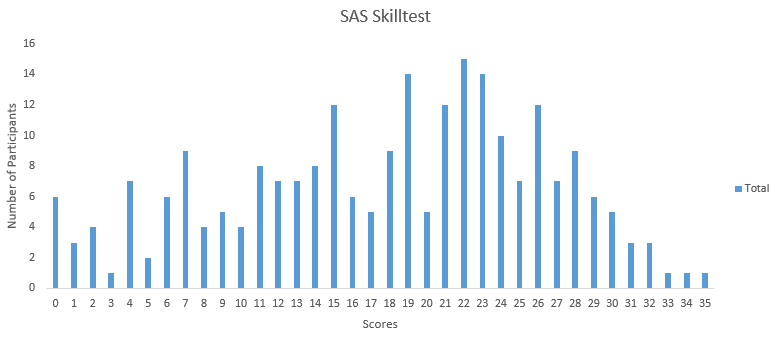You can access your performance here. More than 230 people participated in the skill test and the highest score was 35. Here are a few statistics about the distribution.

Overall distribution

Mean Score: 17.73

Median Score: 19

Mode Score: 22

SAS Learning path and resources – Business Analyst in SAS

Comprehensive Introduction to merging in SAS

Comprehensive guide for Data Exploration in SAS (using Data step and Proc SQL)

Q1) Which one of the following is the value of the variable c in the output data set?

```data work.one;
a = 2;
b = 3;
c = a ** b;
run;```

A) 6
B) 9
C) 8
D) None of the above

Solution: (C)

** is an exponential operator.

so c= a **b = 2**3 = 8

Q2) Which one of the following statement can’t be part of “PROC FREQ”?

A) OUTPUT

B) WEIGHT

C) SET

D) Tables

E) None of the above

Solution: (C)

Look at the syntax of PROC FREQ, there is not SET statement required.

```PROC FREQ <options> ;
BY variables ;
EXACT statistic-options </ computation-options> ;
OUTPUT <OUT=SAS-data-set> options ;
TABLES requests </ options> ;
TEST options ;
WEIGHT variable </ option> ;
RUN;
```

Q3) We have submitted the following PROC SORT step, which generates an output data set.

```proc sort data = AV.employee out = employee;
by Designation;
run;```

In which library is the output data set stored?

A) Work

B) AV

C) SASHELP

D) SASUSER

Solution: (A)

If we are not providing library name explicitly then it will automatically refer to temporary library “WORK”.

Question Context Q4 – Q7
Below are the two tables: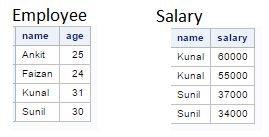Q4) How many variables would be in table “AV” after executing the below SAS program?

```data AV;
merge Employee Salary;
by name;
totsal + salary;
run;

```

A) 3

B) 4

C) 5

Solution: (B)

If we are using any variable name within data step program it will automatically get created in output data set. Here, Three unique variables in both the tables are “name”, “age”, “salary” and one more variable created within dataset “totsal”.

Q5) After executing below SAS program, how many observations would be AV dataset?

```data AV;
merge employee (in=ine) salary(in=ins);
by name;
run;```

A) 4

B) 2

C) 1

D) 6

Solution: (D)

Above you look at input data sets, there is a one-to-many relationship between Employee and Salary. To know more about merging in SAS, click here.

Q6) After executing below SAS program, how many observations would be in AV dataset?

```data AV;
merge employee (in=ine) salary(in=ins);
by name;
if ins=0;
run;```

A) 4

B) 2

C) 1

D) 6

Solution: (B)

Here, we are talking about “in” variables and look at the below table to understand the value of in variables: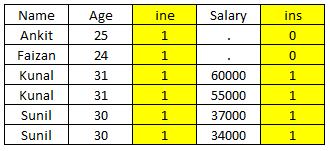In this program, we are looking for observations where ins = 0 which means that “name” values not available in table “Salary”. In above table, you can see that only two records satisfy that criteria.

Q7) Which one of the following command will help us to rename the column “Salary” to “Compensation” of table “Salary”?

A.

```Data Salary (Rename (Salary = Compensation));
Set Salary;
run;```

B.

```Data Salary (Rename = (Salary = Compensation));
Set Salary;
run;```

C.

```Data Salary (Rename = (Salary == Compensation));
Set Salary;
run;```

D. None of the above

Solution: (B)

Syntax to rename variable(s) in SAS is:

RENAME = (Old_Var1 = NewVar1 Old_Var2=New_Var2 Old_Var3=New_Var3 …)

Q8) Which of the following statements is not correct about the program shown below?

```data AV;
do year=2000 to 2004;
Capital+5000;
capital+(capital*.10);
output;
end;
run;```
1. The OUTPUT statement writes current values to the dataset immediately
2. In this case, last value for Year in the new data set is 2005
3. The OUTPUT statement overrides the automatic output at the end of the DATA step
4. The DO loop performs 5 iterations

Solution: (B)

In above program, we are writing to output dataset before END statement which means it will not write last value 2005 to output dataset so last value would be 2004. If we remove OUTPUT statement, last value would be 2005.

Q9) How can you limit the variables written to output dataset in DATA STEP?

1. DROP
2. KEEP
3. RETAIN
4. VAR
5. Both A or B
6. Both A, B or C

Solution: (E)

Both DROP and KEEP can be used to  limit the variables in the dataset.

• The DROP= option tells SAS which variables you want to drop. If you place the DROP= option on the SET statement, SAS drops the specified variables when it reads the input data set and if you place the DROP= option on the DATA statement, SAS drops the specified variables when it writes to the output data set.
• The KEEP= option tells SAS which variables you want to keep. If you place the KEEP= option on the SET statement, SAS keeps the listed variables when it reads the input data set. On the other hand, if you place the KEEP= option on the DATA statement, SAS keeps the specified variables when it writes to the output data set.

Q10) Which of the following statements are used to read delimited raw data file and create an SAS data set?

1. DATA and SET
2. DATA, SET and INFILE
3. DATA, SET and INPUT
4. DATA, INFILE and INPUT

Solution: (D)

SET can not be used to read raw data files. SET is used to read data from one or more SAS dataset.

Question context Q11 – Q12

Below is the data from a csv file “Emp.csv”

Employee id,Gender,Name,DOB,Location,Salary,ManagerEmp ID

This dataset is about company employee

101,M,John,12/1/1995,Delhi,350000,101

102,F,Sangeeta,7/4/1980,Delhi,450000,103

103,F,Mary,3/5/1973,Mumbai,500000,101

104,M,Richard,6/25/1975,Mumbai,750000,101

105,M,Fredrick,8/20/1990,Delhi,320000,101

And, following code is used to read the filenamed EMP.

Q11) What will be the output if we run the below SAS statements to read “emp.csv” file?

```data WORK.EMP;
infile'C:\AV\Skilltest\Emp.csv'dlm=','  ;
input
Employee_id \$
Gender \$
Name \$
DOB
Location \$
Salary
Manager_Emp_ID;
run;```

D. None of the above

Solution: (C)

INFILE statement start reading a file from first line of CSV and it can be header row also so we need to mention start row explicitly.

Q12) Which option will be added to infile statement to read a dataset from the record with employee name “John”?

```data WORK.EMP;
infile'C:\AV\Skilltest\Emp.csv'dlm=','  ;
input
Employee_id \$
Gender \$
Name \$
DOB
Location \$
Salary
Manager_Emp_ID;
run;```

A.  rows=3

B.  option= 3

C.  firstobs=2

D.  start=3

E.  Start=2

F.  firstobs=3

Solution: (F)

FIRSTOBS option can be used to explicity mention the start row to read. In above table, first row is representing header, second row about table and data set is starting with third row.

Q13) Below SAS statements are used to read file “Emp.csv” from third record of csv file.

Code:

```data WORK.EMP;
infile'C:\AV\Skilltest\Emp.csv'dlm=',' DSD firstobs =3;
input
Employee_id \$
Gender \$
Name \$
DOB
Location \$
Salary
Manager_Emp_ID;
run;```

Output: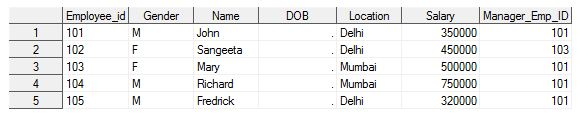Now, which statement we should add to the above code to read date column “DOB” correctly?

A.  Date 360

B.  In-format and format

C.  Both A and B

D.  None of the above

Solution: (B)

To read date column, we need to explicitly mention the format type of date and that can be done using INFORMAT and FORMAT  statements.

Question Context 14

In the snapshot below, you can see that variable “Avg” is in character format.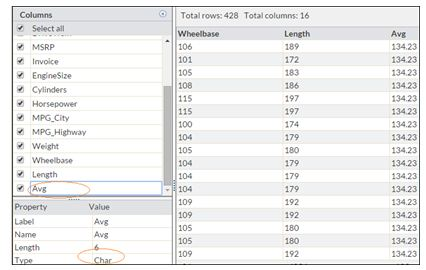Q14) Which of the following statement will help to convert “Avg” to numeric format?

A.  Input(Avg, 5.2)

B.  PUT(Avg,5.2)

C.  INT(Avg,5.2)

D.  Both A and C

Solution: (A)

INPUT() and PUT() are conversion function in SAS. INPUT() is used to convert text to a number whereas PUT() to convert the number to text.

Question Context 15 – 17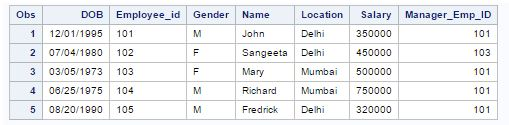Q15) The following SAS program is run on the above table “Emp”

```proc print data = emp;
where Name like '_R%';
run;```

How many records will it print?

A.  1

B.  2

C.  3

D.  None of the above

Solution: (D)

Like operator acts as case sensitive and in above table there is no-one whose second character of the name is capital R.

Q16) Which of the following statement will calculate the age of each employee as on 05-Feb-2017?

A.

```data emp;
set emp;
Age = yrdif(DOB,'05Feb2017'd,'Actual');
run;```

B.

```data emp;
set emp;
Age = yrdif(DOB,'05Feb2017','Actual');
run;```

C.

```data emp;
set emp;
Age = yrdiff(DOB,'05Feb2017','Actual');
run;```

D. None of the above

Solution: (A)

In SAS, date string is always followed by “d” to act as date.

Q17) If you submit the following program on above data set, which variables appear in table “Emp”?

```data emp(drop=Manager_EMP_ID Salary);
set emp (keep=Manager_EMP_ID Employee_ID Salary);
if Manager_Emp_ID=101 and Salary >45000;
Age = yrdif(DOB,'05Feb2017'd,'Actual'd);
run;```

A.  Employee_Id, Gender, Name, Location, Salary, DOB, Manager_Emp_ID

B.  Employee_Id, Gender, Name, Location, Salary, DOB, Manager_Emp_ID, Age

C.  Employee_ID

D.  Employee_ID, Age

E.  Employee_ID, Age, DOB

Solution: (D)

We have only three variables from input dataset “Manager_EMP_ID”,”Employee_ID”, “Salary” and two new variables introduced “DOB” and “Age”. In Data statement, we have dropped two (Manager_EMP_ID” and “Salary”) out of these five variables. Now variables in output dataset “Employee_ID”, “Age”, and “DOB”.

Question context 18

Below is the csv file “class.csv” for marks of students in different subjects:

Name,Gender,Location,English,Maths,Hindi,Sanskrit

Mohan,M,Banglore,50,60,70,80

Ramesh,M,Banglore,45,50,65,89

John,M,Washington,68,,,88

Kathy,F,Washington,89,55,85,83

George,M,Washington,43,45,95,84

Lisa,F,Washington,76,85,,86

Venkat,M,Banglore,68,90,78,92

Srimohan,M,Banglore,59,56,80

Preet,F,Banglore,81,95,85,96

Lindsy,F,Washington,66,75,78,82

Below code is used to read the file class.csv into a SAS dataset table named class.

```data WORK.class;
infile'C:\AV\Skilltest\ClassScore.csv'dlm=','firstobs=2;
input
Name \$
Gender \$
Location \$
English
Maths
Hindi
Sanskrit;
run;```

Above code gives the below output: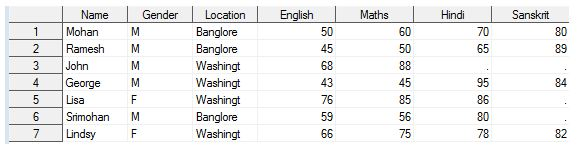Q18) In the above output, you can see following issues:

• Marks are not under right column heading like John marks in”Sanskrit” has shifted to “Maths”
• The total number of observation is 7 only.

Which of the following command can be used with “infile” statement to remove these errors?

A.  MISSING

B.  MISSOVER

C.  DSD

D.  Both A and C

E.  Both B and C

Solution: (E)

Whenever a read a delimited file using infile statement and if the file has two or more delimiter together (n value between them) or last column data is missing then it takes the next possible value as an input for that column. And, the next possible value can be other column data of same row or next line also.

Now, to avoid these reading issues, we use DSD to prevent reading from next column of the same row and MISSOVER for next line or observation.

Question context 19

Below is the table “Class”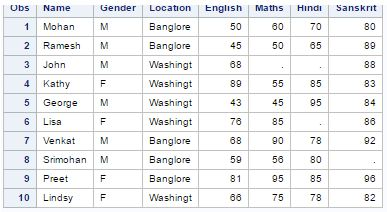Q19) Which of the following command will find the number of missing marks in all variables of table “Class”.

A.

```proc means data=class N;
run;```

B.

```proc means data=class N NMISS;
run;```

C.

```proc means data=class SUM N;
run;```

D.  Both B and C

Solution: (B)

Options with PROC MEANS:

• N: Number of observations with a non-missing value of the analysis variable
• MEAN: Mean (Average) of the analysis variable’s non-missing values
• STD: Standard Deviation
• MAX: Largest (Maximum) Value
• MIN: Smallest (Minimum) Value
• NMISS: Number of missing Values

Q20) Which of the following command will help to impute the missing value of column “Hindi” with average marks of “Hindi”?

A)

```Proc SQL;
Create table temp as Select *, mean(Hindi)  as avg_score from Class;
quit;

Data class (drop= Hindi avg_score Rename=(Hindi_2=Hindi));
Set temp;
If Hindi=. Then Hindi_2=avg_score;
Else Hindi_2=Hindi;
run;```

B)

```Proc SQL;
Create table temp as Select *, mean(Hindi)  asavg_score from Class;
quit;

Data class (drop= Hindi avg_score Rename=(Hindi_2=Hindi));
Set class;
If Hindi=. Then Hindi_2=avg_score;
Else Hindi_2=Hindi;
run;```

C) Both A and B

D)  None of the above

Solution: (A)

In the first option, we are creating a variable avg_score in the table temp and then using this table data in data step to input missing values of HINDI whereas in option second, we are using table class as an input data set for data step.

Question Context 21 – 24

 Table-1 Product_ID Location Proposed_Booking_Date Qty_MT Discount_Dollar A201 Delhi_NCR 12-Jan-17 4 10 A304 Chennai 12-Jan-17 5 20 A205 Mumbai 15-Jan-17 2 4 C406 Delhi_NCR 17-Jan-17 8 5 C203 Delhi_NCR 20-Jan-17 7 1 Z404 Mumbai 15-Jan-17 6 12

 Table-2 Product_ID Location Proposed_Booking_Date Qty_MT Discount_Dollar A210 Mumbai 14-Jan-17 10 10 A310 Mumbai 14-Jan-17 8 20 A354 Delhi 18-Jan-17 5 4 C406 Delhi 17-Jan-17 8 5 C203 Delhi 20-Jan-17 7 1 Z514 Delhi 18-Jan-17 10 15

 Table 3 Date Dollar Rate 12-Jan-17 67.1 14-Jan-17 67.2 15-Jan-17 66.6 17-Jan-17 67.2 18-Jan-17 66.5 20-Jan-17 66.8

Q21) Which of the following statements can be used to append the Table-1 and Table-2 having a unique value of Product_ID?

A.

```data work.merge_table NODUPKEY;
set table1 table2;
run;```

B.

```data work.merge_table;
set table1 table2;
run;

PROC SORT DATA = merge_table OUT = merge_table NODUPKEY;
by Product_ID;
run;```

C.

```data work.merge_table;
set table1 table2 nodupkey;
run;

PROC SORT DATA = merge_table OUT = merge_table;
by Product_ID ;
run;```

D.  none of the above

Solution: (B)

To remove duplicate records based on a variable or multiple variables, we use NODUPKEY with PROC SORT or FIRST./ LAST. option to remove duplicate records. For more detail on removing duplicate records, you can refer this link.

Q22) With cash crunch (due to demonetization) the company decided to advance the proposed booking date by 2 months (keeping the day intact). Which of the below SAS formula can be used to advance the date?

A.

```data work.av_date;
set work.merge_table;
proposed_booking_date1=put(intnx('month',proposed_booking_date,day),date9.);
run;```

B.

```data work.av_date;
set work.merge_table;
proposed_booking_date1=put(intnx('month',proposed_booking_date,2,'s'),date9.);
run;```

C.

```data work.av_date;
set work.merge_table;
proposed_booking_date1=put(intnx('month',proposed_booking_date,sameday),date9.);
run;```

D.

```data work.av_date;
set work.merge_table;
proposed_booking_date1=put(intnx('month',proposed_booking_date,1),date9.);
run;```

Solution: (B)

Look, at the syntax of INTNX() function:

INTNX ( interval, from, n < , alignment > ) ;

The arguments to the INTNX function are as follows:

interval: is a character constant or variable that contains an interval name

from: is a SAS date value (for date intervals) or datetime value (for datetime intervals)

n: is the number of intervals to increment from the interval that contains the from value

alignment: controls the alignment of SAS dates, within the interval, used to identify output observations. Allowed values are BEGINNING, MIDDLE, END, and SAMEDAY/S.

In the second option, you can see that we have used the similar syntax to advance the date value by 2 months.

Q23) If the following code will run, what will be the output?

```data table_A (Drop = Location);
merge table1(in=Proposed_Booking_Date) table3(in=Date);
if Proposed_Booking_Date;
if Date then Discount_INR=Discount_Dollar*Dollar_Rate;
run;

```

A.

 Product_ID Proposed_Bokking_Date Qty_MT Discount_Dollar Dollar_Rate Discount_INR A201 12-Jan-17 4 \$10 67.1 671 A304 12-Jan-17 5 \$20 67.2 1344 A205 15-Jan-17 2 \$4 66.6 266.4 C406 17-Jan-17 8 \$5 67.2 336 C203 20-Jan-17 7 \$1 66.5 66.5 Z404 15-Jan-17 6 \$12 66.8 801.6

B.

 Product_ID Proposed_Bokking_Date Date Qty_MT Discount_Dollar Dollar_Rate Discount_INR A201 12-Jan-17 12-Jan-17 4 \$10 67.1 671 A304 12-Jan-17 12-Jan-17 5 \$20 67.2 1344 A205 15-Jan-17 15-Jan-17 2 \$4 66.6 266.4 C406 17-Jan-17 17-Jan-17 8 \$5 67.2 336 C203 20-Jan-17 20-Jan-17 7 \$1 66.5 66.5 Z404 15-Jan-17 15-Jan-17 6 \$12 66.8 801.6

C.

 Product_ID Qty_MT Discount_Dollar Dollar_Rate Discount_INR A201 4 \$10 67.1 671 A304 5 \$20 67.2 1344 A205 2 \$4 66.6 266.4 C406 8 \$5 67.2 336 C203 7 \$1 66.5 66.5 Z404 6 \$12 66.8 801.6

Solution: (C)

“IN” variable does not appear in output dataset. Here, “Proposed_Booking_Date” and “Date” are “IN” variables and we have dropped the variable “Location” in data step.

Q24) In Table-2, Location name ‘Delhi’ has been wrongly put, need to replace this with ‘Delhi_NCR’. Which of the following code will complete this task?

A.

```data t2;
set TABLE2;
if Location="Delhi" then Location="Delhi_NCR";
run;```

B.

```data t2;
format location \$10.;
set TABLE2;
if Location="Delhi" then Location="Delhi_NCR";
run;```

C.

```data t2;
length Location \$10;
format location \$10.;
set TABLE2;
if Location="Delhi" then Location="Delhi_NCR";
run;```

D. Both B and C

E. Both A and B

F. None of the above

Solution: (D)

The length of field “Location” in table2 is 8 so first we need to change the format of “Location”. Here in both options B and C, we have changed the length of field “Location”.

Q25) [ True | False] Value of First. BY-variable and Last. By-variable can be same.

A.  True

B.  False

Solution: (A)

Yes, it is possible. In case of one unique value for BY variable then this record is the first and last record as well.

Q26) Which is pointer control used to read multiple records sequentially?

A.  @n

B.  +N

C.  /

D.  All of the above

Solution: (C)

You can use one or more forward slash (/) line pointer controls in your INPUT statements to tell SAS to advance to a new record before reading the next data value.

Question Context 27 – 30

Table 5

 Loan_ID Gender Name Dependents Education LoanAmount Property_Area Loan_Status LP001002 Male Dr.Kunal 0 Graduate 145 Urban Y LP001003 Male Mr. Faizan 1 Graduate 128 Rural N LP001005 Female Miss. Swati 0 Graduate 66 Urban Y LP001006 Female Miss. Deepika 0 Not Graduate 120 Urban H LP001008 Male Master Ankit 0 Graduate 141 Urban Y

NOTE: The dataset has been loaded in SAS and table name is table5.

Q27) Categorical column may contain more than two distinct values. For example, “Married” has two values, “Yes” and “No”. How will you find all the distinct values present in the column “Education”?

A.

```proc freq data=Table5;
tables Education;
run;```

B.

```proc means data=Table5;
var Education;
run;```

C. Both A and B

D.  None of the above

Solution: (A)

Proc Means is used to look at the frequency distribution of categories of a categorical variable whereas PROC Means used to explore continuous variables.

Q28) How will you create an extra column “Salutation”?

 Loan_ID Gender Name Salutation Dependents Education LoanAmount Property_Area Loan_Status LP001002 Male Dr.Kunal Dr 0 Graduate 145 Urban Y LP001003 Male Mr. Faizan Mr 1 Graduate 128 Rural N LP001005 Male Miss. Swati Miss 0 Graduate 66 Urban Y LP001006 Male Miss. Deepika Miss 0 Not Graduate 120 Urban H LP001008 Male Master Ankit Master 0 Graduate 141 Urban Y

A.

```data Table5;
set Table5;
Salutation = scan(name, 1);
run;```

B.

```data Table5;
set Table5;
Salutation = scan(name, -1);
run;```

C.

```data Table5;
set Table5;
Salutation = scan(name, 0);
run;```

D

```data test2;
set Table5;
Salutation = scan(name, “ ”,1);
run;```

E

```data test2;
set Table5;
Salutation = scan(name, “.”,1);
run;```

Solution: (A)

Below is the syntax of function SCAN:

SCAN(string, count_words)

String: A constant string or variable have a string value

Count: is a nonzero numeric constant, variable, or expression that has an integer value that specifies the number of the word in the character string that you want SCAN to select

• If count is positive, SCAN counts words from left to right in the character string.
• If count is negative, SCAN counts words from right to left in the character string.

In above question, we need to extract the first word of string so value of count would be 1 and string variable is “name”.

Q29) Which of the following command will help you to create the below table “AV” (Exactly Similar) based on “Table5”?

AV

 Loan_ID Loan_Status_Y Loan_Status_H Loan_Status_N LP001002 1 0 0 LP001003 0 1 0 LP001005 1 0 0 LP001006 0 0 1 LP001008 1 0 0

A.

```data AV;
Set table5;
if Loan_Status = "Y" then Loan_Status_Y = 1; else Loan_Status_Y = 0;
if Loan_Status = "N" then Loan_Status_N = 1; else Loan_Status_N = 0;
if Loan_Status = "H" then Loan_Status_H = 1; else Loan_Status_H = 0;
run;```

B.

```data AV;
Set table5;
Loan_Status_Y= (Loan_Status = "Y");
Loan_Status_N= (Loan_Status = "N");
Loan_Status_H= (Loan_Status = "H");
run;```

C.  Both A and B

D.  None of the above

Solution: (D)

First of all, here we are creating dummy variables for variable “Loan_Status” (also known as One Hot Encoding). Both Option A and B will create these dummy variables but after execution of both program you will not be able to create exactly similar dataset like AV because it will have more number of variables and the values of dummy variables for “Loan_Status_H” and “Loan_Status_N” is swapped in output table “AV”.

Q30) Which of the following SAS program will help you understand the relationship between two variables “Education” and “Loan_Status”?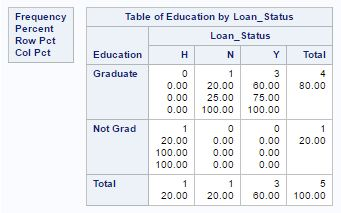A.

```Proc Freq data=table5;
tables Education*Loan_Status;
run;```

B.

```Proc Freq data=table5; ?
tables Education Loan_Status;
run;```

C.

```Proc Univariate data = table5;
var Education Loan_Status;
run;```

D.

```Proc Univariate data = table5;
var Education*Loan_Status;
run;```

Solution: (A)

Above, we are trying to create a two-way table based on two categorical variables “Education” and “Loan_Status”. And to create two-way table, we need to place * in between them. If we will separate the variable name by space then this will create two individual frequency distributions for both the variables.

Q31) [True | Flase] The two programs below will return same output.

Program1

```data AV (Drop= LoanAmount);
set table5;
charge=LoanAmount *0.4;
run;```

Program2

```data AV;
set table5 (Drop= LoanAmount);
charge=LoanAmount *0.4;
run;```

A.   True

B.   False

Solution: (B)

In the first program, we have “LoanAmount” in input data set so there would be values 0.4*LoanAmount in “Charge” column whereas, in the second program, we have dropped the variable “LoanAmount” so the value of column “Charge” would be missing because we do not have variable “LoanAmount”.

Q32) Which of the following statement can be used to accumulate the value of the variable in a Data Step?

A. SET

B. RETAIN

C. UPDATE

D. SUM

Solution: (B)

The RETAIN statement simply copies retaining values by telling the SAS not to reset the variables at the beginning of each iteration of the DATA step. If you would not use retain statement then SAS would reset the variable at the beginning of each iteration

Q33) Given the following SAS error log

```  44   data WORK.OUTPUT;
45     set SASHELP.CLASS;
46     BMI=(Weight*703)/Height**2;
47     where bmi ge 20;
ERROR: Variable bmi is not on file SASHELP.CLASS.
48   run;```

Which of the following step, you will take to correct it?

A.   Replace the WHERE statement with an IF statement

B. Change the ** in the BMI formula to a single *

C.   Change bmi to BMI in the WHERE statement

Solution: (A)

We can not apply WHERE on derived or calculated variable(s) so we should use IF for subsetting.

Q34) Which of the following statement can be used to transpose table “Base” to table “Transposed”?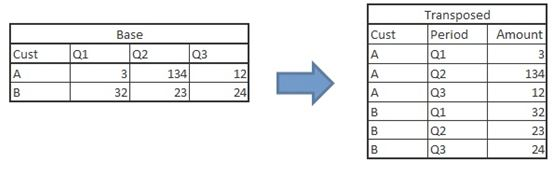A.

```data transposed;
set base;
array Qtr{3} Q:;
do i = 1 to 3;
Period = cat('Qtr',i);
Amount = Qtr{i};
output;
end;
drop Q1 Q2 Q3 i;
if Amount ne .;
run;```

B.

```proc transpose data = base out = transposed
(rename=(Col1=Amount) where=(Amount ne .)) name=Period;
by cust;
run;```

C.       Both A and B

D.     None of the above

Solution: (C)

Both program can be used to transpose the data set, One is array approach whereas in second method, we are using PROC Transpose.

Q35) [True | False] “Where” and “IF” always returns the same result.

A) True

B) False

Solution: (B)

One of the scenarios, we have discussed in question 35.

Q36) Which of the following PROC can be used to create “Bubble”, “Scatter” and “Histogram”?

A. PROC SGPLOT

B. PROC UNIVARIATE

C. PROC PLOT

D. None of the above

Solution: (A)

PROC SGPLOT can be used to create all above-mentioned charts.

Question Context 37 – 38

Table6

 Month Product1 Product2 Product3 Jan 30 38 39 Feb 35 43 47 Mar 68 70 78 Apr 18 26 26 May 25 31 33 Jun 29 36 40 Jul 34 38 47 Aug 34 37 43 Sep 36 43 51 Oct 34 36 43 Nov 32 34 40 Dec 33 43 44

Note: Above table “Table6” is stored in WORK library

Q37) Which of the following command can be used to plot below chart?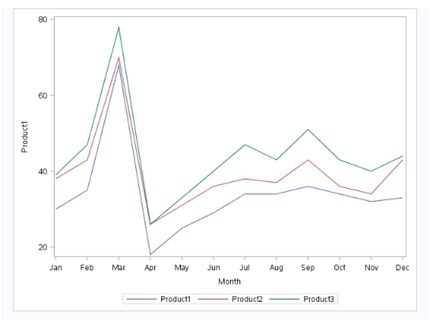A.

```PROC SGPLOT DATA = Table6;
SERIES X = Month Y = Product1;
SERIES X = Month Y = Product2;
SERIES X = Month Y = Product3;
run;```

B.

```PROC SGPLOT DATA = Table6;
by Month;
Var Product1 Product2 Product3;
run;```

C.

```PROC SGPLOT DATA = Table6;
Line Month;
Var Product1 Product2 Product3;
run;```

D. None of the above

Solution: (A)

Above, we are creating three series of line in a single chart and we don’t have any “Line” and “BY” statements in PROC SGPLOT.

Q38) Which of the following command can be used to plot below chart (Below Product1 is represented on x-axis, Product2 on y-axis and Product3 as the size of bubble)?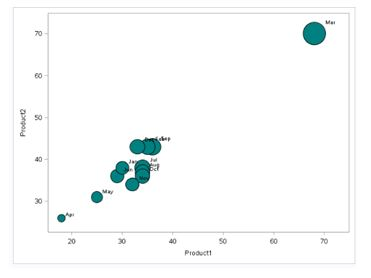A.

```proc plot data = Table6;
scatter X=Product1 Y=Product2 size= Product3
/fillattrs=(color = teal) datalabel = Month;
run;```

B.

```proc sgplot data = Table6;
bubble X=Product1 Y=Product2 size= Product3
/fillattrs=(color = teal) datalabel = Month;
run;```

C.

```proc sgplot data = Table6;
scatter X=Product1 Y=Product2 size= Product3
/fillattrs=(color = teal) datalabel = Month;
run;```

D.

```proc chart data = Table6;
scatter X=Product1 Y=Product2 size= Product3
/fillattrs=(color = teal) datalabel = Month;
run;```

Solution: (B)

In bubble chart, we have three variables to visualize. One on x-axis, second one on y-axis and last one as size of bubble. We can create Bubble chart in SAS using PROC SGPLOT with Bubble statement.

Question Context 39 – 40

Below is the table of product inventory (SAS data set name is “Table7”)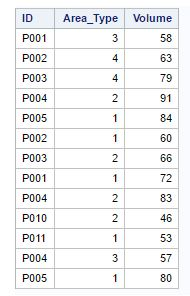Q39) Which of the following SAS program will remove the duplicate observation(s) of “ID” and “Area_Type”. And, remove observation having the lower magnitude of variable “Volume”?

A.

```Proc Sort data=table7;
by ID Area_Type Descending Volume;
run;
Proc SORT Data=table7 out=table8 nodupkey;
by ID Area_Type;
run;```

B.

```Proc Sort data=table7;
by ID Area_TypeVolume Descending;
run;
Proc SORT Data=table7 out=table8 nodupkey;
by ID Area_Type;
run;```

C.

```Proc SORT Data=table7 out=table8 nodupkey;
by ID Area_TYPE Volume Descending;
run;```

D. Both B and C

Solution: (A)

The basic problem with Option B and C is, “Descending” option is appearing after the variable name which is not the right syntax. In option A, we are first sorting the data set based on “ID”, “Area_Type” and “Volume” (Descending) then again writing a PROC SORT to remove duplicate records based on “ID” and “Area_Type”.

Q40) Which of the following program will help to bin the variable volume (Adding one more variable to “Table7”, “Volume_Bucket”)?

A.

```Data table7;
set table7;
select (Volume);
when (le 30)     Volume_Bucket="A";
when (le 60)     Volume_Bucket="B";
otherwise        Volume_Bucket="C";
end;
run;```

B.

```Data table7;
set table7;
if Volume < 30 then Volume_Bucket ="A";
Else if Volume <60 then Volume_Bucket="B";
Else Volume_Bucket="C";
run;```

C. Both A and B

D. None of the above

Solution: (B)

Select statement works with exact value, it does not compare like greater than or less than so here IF statement will do the task.

## End Notes

I hope you enjoyed taking the test and found the solutions helpful. The test focused on conceptual as well as practical knowledge of Base Programming in SAS

I tried to clear all your doubts through this article, but if we have missed out on something then let us know in comments below. Also, If you have any suggestions or improvements you think we should make in the next skill test, you can let us know by dropping your feedback in the comments section.

## Learn, compete, hack and get hired!

You can also read this article on our Mobile APP•Nisha says:

In Q8 how will the do loop perform 15 iterations?
According to me A and C are correct statements, therefore answer should be B and D

•Sunil Ray says:

Hi Nisha,

do loop will iterate 5 times and last year value would be 2005 also but data step will not write this to output data set because we have output statement within loop. Which means it will not write last value 2005 to output dataset so last value would be 2004. If we remove OUTPUT statement, last value would be 2005.

•Geetika says:

The loop will iterate 5 times, so the last statement ‘The DO loop performs 15 iterations’ is also not true and hence the answer to this. Can you please advise?

•Sunil Ray says:

It was a typo here in the solution article. It is actually 5 times. Thanks for highlighting it.

•Lokeshastro says:

Hi Sunil,

First of all thanks for a wonderful test.
I have checked all the question and found there are some mistakes ( may be typo).
Here are those..

Q6 has wrong answers, as in explanation you have given the correct answer (2 obs) but the option is showing the correct ans is 4, which is wrong.

Q8: In this question there are 2 incorrect options given in the options, first as you said B and the other one is D , as this loop will do 5 iterations not 15

Q18: For the given data only DSD will read the file properly .. however in any other raw data as well if the delimiters are placed properly then we dont need to use missover with DSD as it takes a single delimiter as missing for the first and last variable.
for eg:
data test;
infile datalines dsd;
input N1 N2 N3;
datalines;
,2,
1,2,3
1,2,
,,3
,,
1,,3
;run;

we don’t need to use missover here in this example as it will work, but will fail if there are no delimiter at the end for missing values.

So as per me the correct answer for that question should be option C

Q36: In explanation the correct answer is given SGPLOT. but options displays the wrong answer.

Hope this help, please let me know in case you think i am wrong anywhere.

Hope if you correct the leaderboard again ?

•Sunil Ray says:

Lokesh,

Thanks for your feedback and highlighting the typo.

Question 6 and 36 have a typo about showing the wrong option as an answer in the solution article although you can see that explanation is pointing towards the right answer.

Question 8: Again a typo, option corrected.

Question 18: Yes, you are right, if there is delimiter at the end there is no requirement of MISSOVER,

Regards,
Sunil

•samartha says:

Thank you for taking the time to provide us with your valuable information. We strive to provide our candidates with excellent care.As always, we appreciate you confidence and trust in us.